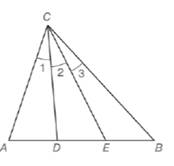Chapter 5.6, Problem 21EElementary Geometry For College St...

7th Edition
Alexander + 2 others
ISBN: 9781337614085

Solutions

Chapter
SectionElementary Geometry For College St...

7th Edition
Alexander + 2 others
ISBN: 9781337614085
Textbook Problem

In Δ A B C , ∠ A C B is trisected by C D → and C E → so that ∠ 1 ≅ ∠ 2 ≅ ∠ 3. Write two different proportions that follow from this information.To determine

To write:

Two proportions that follow from the given information.

Explanation

Given:

In ΔABC, ACB is trisected by CD and CE so that 123.

Theorem used:

Angle bisector theorem:

If a ray bisects one angle of a triangle, then it divides the opposite side into segments whose lengths are proportional to the lengths of the two sides that form the bisected angle.

Calculation:

Consider the given figure.

Still sussing out bartleby?

Check out a sample textbook solution.

See a sample solution

The Solution to Your Study Problems

Bartleby provides explanations to thousands of textbook problems written by our experts, many with advanced degrees!

Get Started

x+1x1=5

Applied Calculus for the Managerial, Life, and Social Sciences: A Brief Approach

Evaluate the definite integral. 0/6sintcos2tdt

Single Variable Calculus: Early Transcendentals, Volume I

In Exercises 1-6, simplify the expression. 818

Calculus: An Applied Approach (MindTap Course List)

y=4x2+1 defines y implicitly as a function of x.

Study Guide for Stewart's Single Variable Calculus: Early Transcendentals, 8th